Electron. J. Differential Equations, Vol. 2017 (2017), No. 187, pp. 1-18.

### Second-order boundary estimate for the solution to infinity Laplace equations Ling Mi

Abstract:
In this article, we establish a second-order estimate for the solutions to the infinity Laplace equation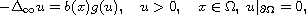where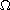is a bounded domain in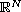,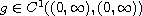,is decreasing on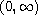with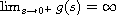and g is normalized regularly varying at zero with index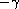(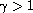),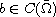is positive in, may be vanishing on the boundary. Our analysis is based on Karamata regular variation theory.

Submitted December 18, 2016. Published July 24, 2017.
Math Subject Classifications: 35J55, 35J60, 35J65.
Key Words: Infinity Laplace equation; second order estimate; Karamata regular variation theory; comparison functions.

Show me the PDF file (289 KB), TEX file for this article.Ling Mi College of Mathematics and Statistics Linyi University Linyi, Shang Dong 276005, China email: mi-ling@163.com, miling@lyu.edu.cn